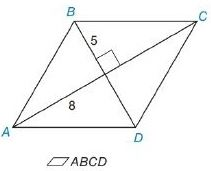Chapter 8.2, Problem 15EElementary Geometry For College St...

7th Edition
Alexander + 2 others
ISBN: 9781337614085

Solutions

Chapter
SectionElementary Geometry For College St...

7th Edition
Alexander + 2 others
ISBN: 9781337614085
Textbook Problem

In Exercises 13 to 18, find the area of the given polygon.To determine

To Find:

Area of the rhombus.

Explanation

Formula Used:

The area A of a rhombus whose diagonals has lengths d1 and d2 is given by A=12d1d2.

Diagonals of a rhombus are perpendicular bisector of each other.

Calculation:

It is given that AB¯=BC¯. Let the diagonals meet at O.

Also given that BO=5 and AO=8.

In rhombus, opposite sides are parallel and all sides are in equal length.

Therefore, AO=OC=8 and BO=OD=5

Still sussing out bartleby?

Check out a sample textbook solution.

See a sample solution

The Solution to Your Study Problems

Bartleby provides explanations to thousands of textbook problems written by our experts, many with advanced degrees!

Get Started

Solve the equations in Exercises 126. 2x3x2x3x+1=0

Finite Mathematics and Applied Calculus (MindTap Course List)

In Problems 14-19, find the value of each sum. 16.

Mathematical Applications for the Management, Life, and Social Sciences

Given: AEBDEC AEDE Prove: AEBDEC

Elementary Geometry for College Students

List the major sections of an APA-style report in order of appearance.

Research Methods for the Behavioral Sciences (MindTap Course List)